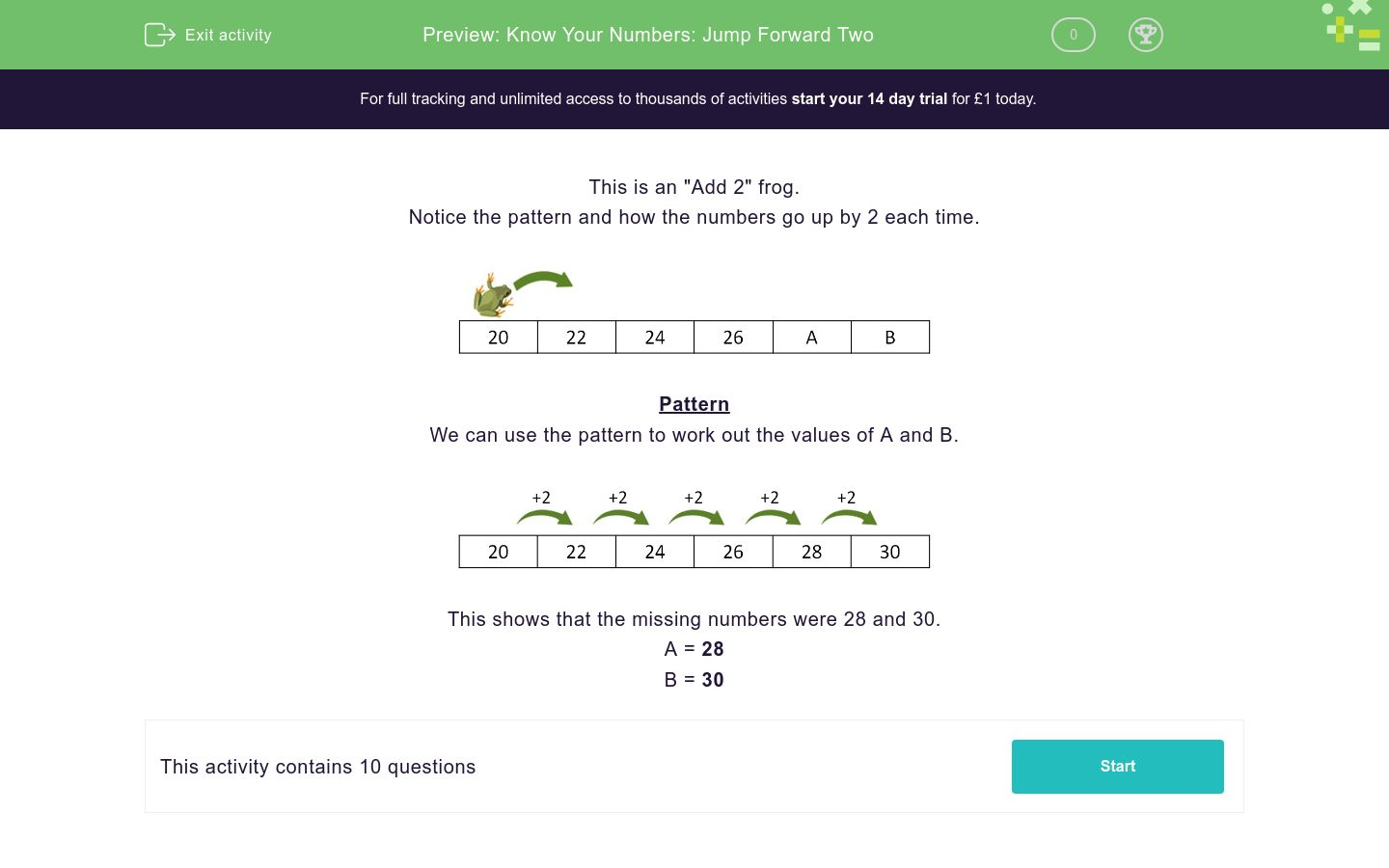# Know Your Numbers: Jump Forward Two

In this worksheet, students find the missing numbers in a sequence that goes up in twos.Key stage:  KS 1

Curriculum topic:   Number: Number and Place Value

Curriculum subtopic:   Count in Steps (2, 3, 5 and 10)

Difficulty level:### QUESTION 1 of 10

This is an "Add 2" frog.

Notice the pattern and how the numbers go up by 2 each time.Pattern

We can use the pattern to work out the values of A and B.This shows that the missing numbers were 28 and 30.

A = 28

B = 30

This is an "Add 2" frog.

Work out the values of A and B.16 18 20 22 A B
 A B 24 28 22 26

This is an "Add 2" frog.

Work out the values of A and B.14 16 18 20 A B
 A B 24 25 22 23

This is an "Add 2" frog.

Work out the values of A and B.16 18 20 A B 26
 A B 24 28 22 26

This is an "Add 2" frog.

Work out the values of A and B.19 21 23 25 A B
 A B 25 27 29 26

This is an "Add 2" frog.

Work out the values of A and B.13 15 17 19 A B
 A B 21 24 25 23

This is an "Add 2" frog.

Work out the values of A and B.20 22 24 26 A B
 A B 24 28 22 30

This is an "Add 2" frog.

Work out the values of A and B.18 20 22 24 A B
 A B 24 28 22 26

This is an "Add 2" frog.

Work out the values of A and B.11 13 15 17 A B
 A B 21 18 19 22

This is an "Add 2" frog.

Work out the values of A and B.13 A 17 19 21 B
 A B 15 23 22 24

This is an "Add 2" frog.

Work out the values of A and B.A 18 20 22 B 26
 A B 24 28 22 16
• Question 1

This is an "Add 2" frog.

Work out the values of A and B.16 18 20 22 A B
 A B 24 28 22 26
• Question 2

This is an "Add 2" frog.

Work out the values of A and B.14 16 18 20 A B
 A B 24 25 22 23
• Question 3

This is an "Add 2" frog.

Work out the values of A and B.16 18 20 A B 26
 A B 24 28 22 26
• Question 4

This is an "Add 2" frog.

Work out the values of A and B.19 21 23 25 A B
 A B 25 27 29 26
• Question 5

This is an "Add 2" frog.

Work out the values of A and B.13 15 17 19 A B
 A B 21 24 25 23
• Question 6

This is an "Add 2" frog.

Work out the values of A and B.20 22 24 26 A B
 A B 24 28 22 30
• Question 7

This is an "Add 2" frog.

Work out the values of A and B.18 20 22 24 A B
 A B 24 28 22 26
• Question 8

This is an "Add 2" frog.

Work out the values of A and B.11 13 15 17 A B
 A B 21 18 19 22
• Question 9

This is an "Add 2" frog.

Work out the values of A and B.13 A 17 19 21 B
 A B 15 23 22 24
• Question 10

This is an "Add 2" frog.

Work out the values of A and B.A 18 20 22 B 26
 A B 24 28 22 16
---- OR ----

Sign up for a £1 trial so you can track and measure your child's progress on this activity.

### What is EdPlace?

We're your National Curriculum aligned online education content provider helping each child succeed in English, maths and science from year 1 to GCSE. With an EdPlace account you’ll be able to track and measure progress, helping each child achieve their best. We build confidence and attainment by personalising each child’s learning at a level that suits them.

Get started